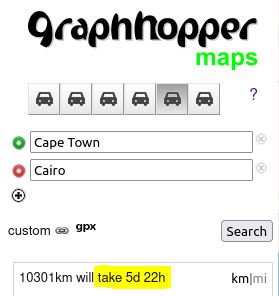# Route time or ETA

Hi all,

In which class is route time or route ETA calculated?The total duration of a path is the sum of the duration of each edge. The duration of each edge is calculated here:

Your main ability to influence the value returned will come from changing the speed of an edge. For example, see here if you’re using the custom model: https://github.com/graphhopper/graphhopper/blob/master/docs/core/custom-models.md#custom-models-by-the-example-of-customizing-speed

If you’re not using the custom model, then the speed of the edges is set in the flag encoder.

Thank you @Sam_Crawford

I have customized the Shortest weighting to include a speedclass tag/encoded value in the formula for my customized Short Easy weighting. Would the ETA and distance both be calculated using calcEdgeWeight = edgeState.getDistance() / speedclass; as below?

``````public class ShortestEasyWeightingSpeedClass extends ShortestWeighting {

private final DecimalEncodedValue xmaxspeedEnc;
private final DecimalEncodedValue xroutingpriorityEnc;
private final DecimalEncodedValue speedClassEnc;
public ShortestEasyWeightingSpeedClass(FlagEncoder encoder) {

super(encoder);

List<EncodedValue> encodedValues  = encoder.getEncodedValues();
System.out.println("Encoded Values:");
for(EncodedValue ev : encodedValues){

}

xmaxspeedEnc = flagEncoder.getDecimalEncodedValue(EncodingManager.getKey(flagEncoder, "x_maxspeed"));
xroutingpriorityEnc = encoder.getDecimalEncodedValue(EncodingManager.getKey(encoder, "x_routing_priority"));
speedClassEnc = encoder.getDecimalEncodedValue(EncodingManager.getKey(encoder, "speedclass"));

}

@Override
public double getMinWeight(double currDistToGoal) {
return currDistToGoal;
}

@Override
public double calcEdgeWeight(EdgeIteratorState edgeState, boolean reverse) {

double speedclass = speedClassEnc.getDecimal(false, edgeState.getFlags());

if (speedclass == 0.0) {
speedclass = 0.125;
} else if (speedclass == 1.0) {
speedclass = 0.25;
} else if (speedclass == 2.0) {
speedclass = 0.375;
} else if (speedclass == 3.0) {
speedclass = 0.5;
} else if (speedclass == 4.0) {
speedclass = 0.625;
} else if (speedclass == 5.0) {
speedclass = 0.75;
} else if (speedclass == 6.0) {
speedclass = 0.875;
} else if (speedclass == 7.0) {
speedclass = 1.0;
}

double calcEdgeWeight = edgeState.getDistance() / speedclass;
return calcEdgeWeight;

}

@Override
public String getName() {
return "short_easy_speedclass";
}
}``````

This topic was automatically closed 90 days after the last reply. New replies are no longer allowed.